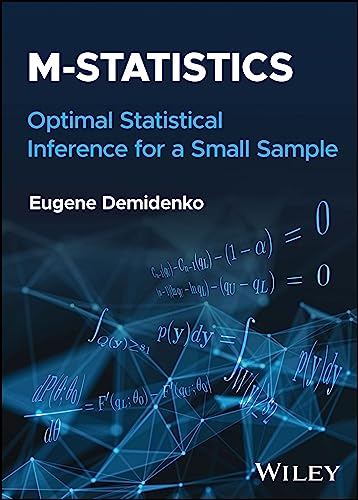# M-statistics: Optimal Statistical Inference for a Small Sample

• Length: 240 pages
• Edition: 1
• Publisher:
• Publication Date: 2023-08-22
• ISBN-10: 1119891795
• ISBN-13: 9781119891796
Description

M-STATISTICS A comprehensive resource providing new statistical methodologies and demonstrating how new approaches work for applications M-statistics introduces a new approach to statistical inference, redesigning the fundamentals of statistics, and improving on the classical methods we already use. This book targets exact optimal statistical inference for a small sample under one methodological umbrella. Two competing approaches are maximum concentration (MC) and mode (MO) statistics combined under one methodological umbrella, which is why the symbolic equation M=MC+MO. M-statistics defines an estimator as the limit point of the MC or MO exact optimal confidence interval when the confidence level approaches zero, the MC and MO estimator, respectively. Neither mean nor variance plays a role in M-statistics theory. Novel statistical methodologies in the form of double-sided unbiased and short confidence intervals and tests apply to major statistical Our new developments are accompanied by respective algorithms and R codes, available at GitHub, and as such readily available for applications. M-statistics is suitable for professionals and students alike. It is highly useful for theoretical statisticians and teachers, researchers, and data science analysts as an alternative to classical and approximate statistical inference.